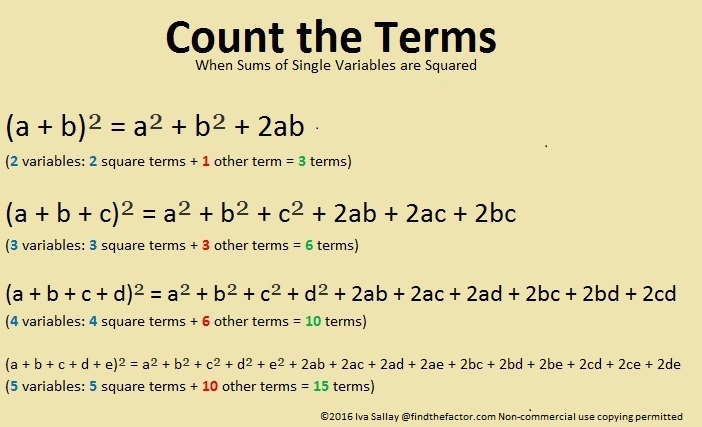780 is the 39th Triangular Number

• 780 is a composite number.
• Prime factorization: 780 = 2 x 2 x 3 x 5 x 13, which can be written 780 = (2^2) x 3 x 5 x 13
• The exponents in the prime factorization are 2, 1, 1, and 1. Adding one to each and multiplying we get (2 + 1)(1 + 1)(1 + 1)(1 + 1) = 2 x 3 x 2 x 2 = 24. Therefore 780 has exactly 24 factors.
• Factors of 780: 1, 2, 3, 4, 5, 6, 10, 12, 13, 15, 20, 26, 30, 39, 52, 60, 65, 78, 130, 156, 195, 260, 390, 780
• Factor pairs: 780 = 1 x 780, 2 x 390, 3 x 260, 4 x 195, 5 x 156, 6 x 130, 10 x 78, 12 x 65, 13 x 60, 15 x 52, 20 x 39, or 26 x 30
• Taking the factor pair with the largest square number factor, we get √780 = (√4)(√195) = 2√195 ≈ 27.92848.There are MANY ways to make factor trees for 780. Here are just three of them:OEIS.org informs us that (7 + 5)(8 + 5)(0 + 5) = 780.

780 is the sum of consecutive prime numbers two different ways:

• 59 + 61 + 67 + 71 + 73 + 79 + 83 + 89 + 97 + 101 = 780 (10 consecutive primes).
• 191 + 193 + 197 + 199 = 780 (4 of my favorite consecutive primes).

26 and 30 are both exactly 2 numbers away from their average 28, so 780 can be written as the difference of two squares:

780 = 26 x 30 = (28 – 2)(28 + 2) = 28² – 2² = 784 – 4.

(It can be written as the difference of two squares three other ways, but I won’t list them here.)

Because 5 and 13 are both factors of 780, it is the hypotenuse of FOUR Pythagorean triangles making each of these equations true:

• 192² + 756² = 780²
• 300² + 720² = 780²
• 396² + 672² = 780²
• 468² + 624² = 780²

780 can be written as the sum of three perfect squares two different ways:

• 26² + 10² + 2² = 780
• 22² + 14² + 10² = 780

780 BASE 10 is palindrome QQ BASE 29. (Q is 26 in BASE 10.) Note that 26(29) + 26(1) = 780.

1 + 2 + 3 + 4 + 5 + 6 + 7 + 8 + 9 + 10 + 11 + 12 + 13 + 14 + 15 + 16 + 17 + 18 + 19 + 20 + 21 + 22 + 23 + 24 + 25 + 26 + 27 + 28 + 29 + 30 + 31 + 32 + 33 + 34 + 35 + 36 + 37 + 38 + 39 = 780, making 780 the 39th triangular number. Since 20 is in the exact middle of that list of numbers, 780 is also the 20th hexagonal number.

Triangular numbers are interesting, but are they good for anything? Here’s one good thing:There are 26 letters in the English alphabet. 39 variables would use the alphabet exactly one and one half times, but that’s okay because we can use upper case letters the second time around:

(a + b + c + . . . + x + y+ z + A + B + C + . . . + K + L + M)² has exactly 780 terms because it has 39 single variables, and 780 is the 39th triangular number.

780 is the 39th triangular number because 39⋅40/2 = 780.

A couple of months ago I saw a fascinating image on twitter. It is a square made with 1001 dots representing the numbers from -500 to +500 with zero in the exact middle. The triangular numbers are represented by the “+” pattern seen here. If we added about 600 more dots to the square, -780 and +780 would also lie on that +.